An Ecosystem of δ-Potentials - IVA Blip: Fermionic or Bosonic?   30th July  2022 HOME "It would be a great step forward to unify in a single picture the gravitational and electromagnetic fields.  Then there would be a worthy completion of the epoch of theoretical physics begun by Faraday and Maxwell..." - A. Einstein, as quoted in "Einstein and the Kaluza-Klein particle" by Joroen van Dongen, arXiv:gr-qc/0009087. "Here we strive for the same goal in a different way." - Th. Kaluza, On the Unification Problem in Physics. "Although incomplete, this result, together with the considerations give here, suggests that the origin of Planck's quantum may be sought just in this periodicity in the fifth dimension." -Oskar Klein, The Atomicity of Electricity as a Quantum Theory Law,  Nature Vol.(18), Page 516, 1926. "The principle of gaining knowledge of the external world from the behaviour of its infinitesimal parts is the mainspring of the theory of knowledge in infinitesimal physics as in Riemann’s geometry, and, indeed, the mainspring of all the eminent work of Riemann, in particular, that dealing with the theory of complex functions." - Hermann Weyl, Space-Time-Matter, Dover Publications, 1952. "The elevation of gauge fields to the level of the gravitational fields is a substantial achievement, but is by no means the end of the story. " - Lochlainn O'Raifeartaigh, The Dawning of Gauge Theory, Princeton Series in Physics, 1997.   *** A quick summary of what we discussed earlier.  Our postulates were: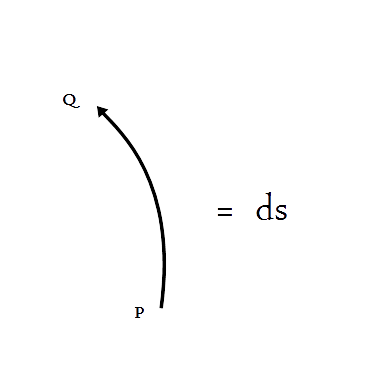Every entity, simultaneously an observer and an object, is in a state of measurement and will continue to measure until the measurement is complete.  Equivalently an observer must arrive at point Q from the point P. Every entity, simultaneously an observer and an object, will measure the curve of the least disorder.  (In j-space, a measurement is represented by the path PQ.)     Next we placed the observer making measurements, ObsM, in S-frame.  And the observer being measured, δ-functions, in S'-frame.  We remind ourselves that Blip the macroscopic world, exists in the exterior region-1 of Kruskal-Szekeres (KS) Coordinates.  And S and S' both, almost always, correspond to the measurements being made in this region.     We reviewed the thermodynamics variables available to us to establish an environment within Blip.  We noted that while the entropy S remained invariant between S and S' in the relativistic limits, the entropy density φ was not.  Another issue was the relationship between mass and energy, defined as: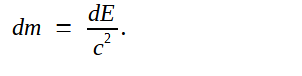While the above relationship worked very well with for the correspondence between the  kinetic energy and change in mass, the incorporation of the potential energy into this correspondence, was a little problematic.  It should be clear however, that both energies combined together were conserved, and so was the rest mass.      So how do we think about a perfectly inelastic interaction and the corresponding change in the mass (combined and individual) of interacting bodies, particularly when the contribution of the kinetic energy component is minimal?1     Earlier we had discussed the problem of mass and information content in j-space, in the context of observers from different information spaces and hence varying measurement capabilities.  However here we have the observer-definitions fixed i.e. ObsM is making measurements in the Region-1.  And the measurements of Obsc define the j-pixel landscape which constitutes the vacuum in the Region-1.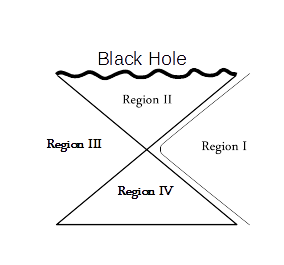Let us bring back the measurement frames, S and S', from the Theory of Special Relativity (SR).2  For the sake of convenience the proper spatial coordinates and the proper time, are denoted by  ξ and τ respectively.  We will also denote the observers in S and S' frames as Obs and Obs' respectively.   Case-1 (Galilean motion, u = 0j u2 << c2): Obs' makes the measurement PQ in S'. Obs measures Obs'-measurements made is S',  in S with complete precision. The rest mass mo can be accurately determined. In this case the parameters (x,t) and (ξ,τ) are identical.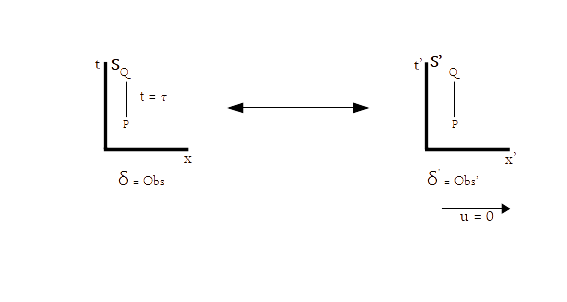Case-2 (Relativistic kinetic motion, u >> 0j u2 ~  c2):      Again Obs' makes the measurement PQ in S'. And Obs measures in S, Obs'-measurements made is S'.   The mass measured in S is given as m =  γ mo$.$    However in this case, (x, t) and (ξ, τ) are different and the path measured in S frame is a geodesic instead of being linear. We remember that the j-space is a resource (Observer's Hamiltonian) based measurement space.  The resources available to an observer, are represented by the observer's Hamiltonian.  When the Obs is making measurements of the Obs', we need to provide a much more powerful Hamiltonian in S-frame, to complete the measurement PQ defined as a linear path in S'.  Thus the measurements made in S-frame, are no longer zero-entropy measurements and as a result the path from P to Q, is no longer linear.3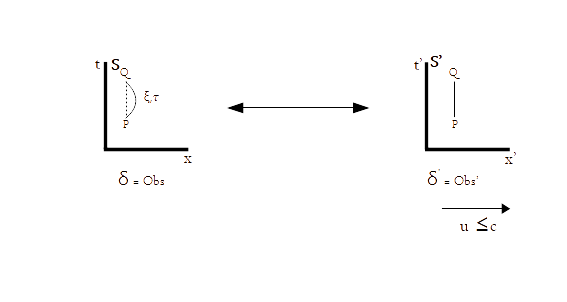Case-3 (Potential Energy >> Kinetic Energy in S', u << 0j ):This case represents an unstable system returning to stability or equilibrium.  The path PQ in S' represents this potential energy based process.  The condition u << 0j  is symbolic of a process where P.E. >> K.E.     Obs' makes the measurement PQ in S'. And Obs measures in S, Obs'-measurements made is S'.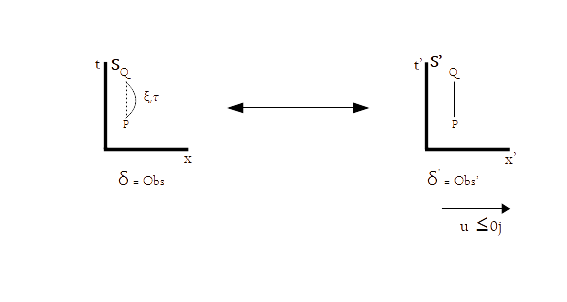Again additional resources are provided to the Obs in S-frame, for example LHC, to complete the measurements. Equivalently we can say that the observer's capability is enhanced by reducing the entropy inherent in the observer's Hamiltonian.  However we do not know how to incorporate this situation into the SR machinery?     How would we determine the value of the measured mass m, in S-frame?     Our subsequent discussion, is on how to consolidate all these cases in SR, at least qualitatively, using the discrete measurement space.   We note that the observer in S-frame does not have adequate resources to measure the rest mass mo of the object in S'-frame, in either Case-2 or Case-3.  However we can make an assumption that in j-space, the observer's measurement capability or equivalently the observer's Hamiltonian, can be enhanced by providing appropriate resources.       Let us consider the relativistic case, Case-2, where u2/c2 ~ 1.  We note that in this case ds = 0j and we called it a zero-entropy measurement.  We were able to define a time-independent constant jML, based on h and c as follows: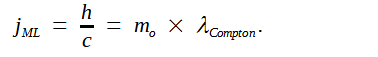When we use Compton wavelength to describe a system (an observer or a delta function), we have moved into de Broglie picture from Schrödinger picture.  Thus de Broglie space (dB-space) represents the topological space for Obs in S-frame.  The dB-space is characterized by the measurements made by Obsc.  Our j-pixels exist in dB-space.     It is not that simple to move into dB-space space for a system which exists in either Galilean or the rest frame S.  It requires that the observer Obs in S-frame has the relativistic capabilities.  By assumption the Hamiltonian for Obs can be enhanced, by adding resources such as Magnetic Field or Kinetic Energy, but that can not be sustained for an epoch.4  Similarly accounting for the case-3 is not straight forward as well.      We note that in j-space, we are in essence comparing the measurements of the observers of different capabilities.  The frames S and S' represent the measurement spaces of the observers inheriting Hamiltonians of different resources.      For example, if the capabilities of the observers in S and S' are represented by η and η', then clearly for the Case-2, η « η'.  However we can further the argument to Case-3 as well, and the condition η « η' applies to Case-2 and Case-3 both.      Similarly the j-pixel vacuum, is the result of the measurements made by the Observer Obsc, whose capability is represented by ηc.  In this case ηc >> η' >> η.   ____________________ 1.  In j-space language, we want to know the physical description, if two δ-functions unique to each other per the measurements of ObsM are interacting with other.  If both δ-functions are singular and not composite, then what would be the mechanism of the interaction?  We are in essence, describing an ideal black hole in the information space. 2. The frames S and S' are inertial only for an infinitesimal slice of time.  For the duration of an epoch S and S' are non-inertial due to the entropy inherent in Obsc measurements. 3. Would the S-frame-geodesic in this case, cross the straight line joining P and Q, before the measurement was complete? 4.  One way to reverse entropy, or in other words to return the geodesic PQ to the linear PQ, is to bring in new information from higher information space, but that is something which is not relevant to this discussion for now.   *** Previous Blogs:   EPR Paradox-I  Nutshell-2015Information on www.ijspace.org is licensed under a Creative Commons Attribution 4.0 International License. Attribution — You must give appropriate credit, provide a link to the license, and indicate if changes were made. You may do so in any reasonable manner, but not in any way that suggests the licensor endorses you or your use. No additional restrictions — You may not apply legal terms or technological measures that legally restrict others from doing anything the license permits. This is a human-readable summary of (and not a substitute for) the license.